Showing first {{hits.length}} results of {{hits_total}} for {{searchQueryText}}{{hits.length}} results for {{searchQueryText}}

There are a few LaTeX packages to create chemistry formulae: chemfig, ochem, streetex, and xymtex. The most intuitive is probably the chemfig package. This article explains how to use the chemfig package to create chemical formulae in LaTeX. Contents 1 Introduction 2 Angles 3 Rings 4 Branches 5 Customizing the formulae 6 Open all the examples 7 Reference guide 8 Further reading Introduction Drawing a molecule consists mainly of connecting groups of atoms with lines. Simple linear formulae can be easily drawn using the chemfig package, as shown in the following example: \documentclass{article} \usepackage{chemfig} \begin{document} \section{Introduction} Writing chemical formulae with chemfig is straightforward. \chemfig{O=H} \end{document}  Open this chemfig example in Overleaf The following image shows the output produced by the example above: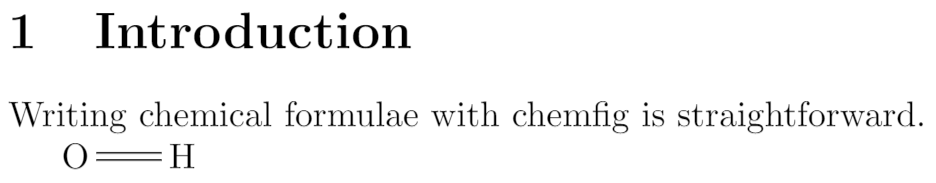The package is imported by \usepackage{chemfig} in the preamble. The command \chemfig{O=H} the draws the molecule. The symbol = determines the type of bond. See the reference guide for a list of bond types. Angles There are several ways to define angles between bonds in molecules. To define chemical formulae you can use units that define the angles \chemfig{A-B-C} Absolute angles \chemfig{A-[:50]B-[:-25]C} Relative angles \chemfig{A-[::50]B-[::-25]C}  Open this chemfig example in Overleaf The following image shows the output produced by the example above: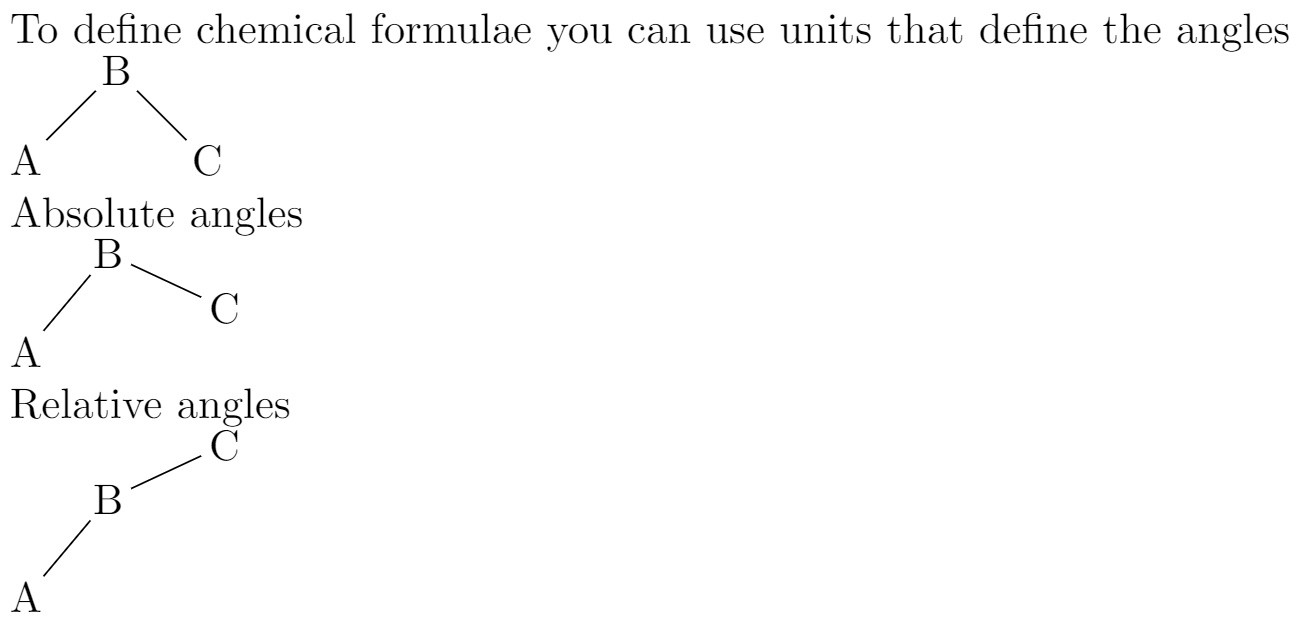Each one of the three commands in the example above uses a different method to determine the angle between bonds. default units: In the command \chemfig{A-B-C} the parameters inside brackets set the angle in special units, each unit equals 45°. Hence in the example the angles are 45° and 315°. absolute units: The angles can be set in absolute units, in the command \chemfig{A-[:50]B-[:-25]C} the parameter inside the brackets represent the angle, in degrees, measured from the horizontal baseline. Negative angles are allowed. relative angles: In the third example \chemfig{A-[::50]B-[::-25]C} the angles are measured from the previous bond, instead of the baseline. Rings The example below presents the syntax to draw regular polygons: Regular polygons: \chemfig{A*5(-B=C-D-E=)} Incomplete rings are also possible: \chemfig{A*5(-B=C-D)}  Open this chemfig example in Overleaf The following image shows the output produced by the example above: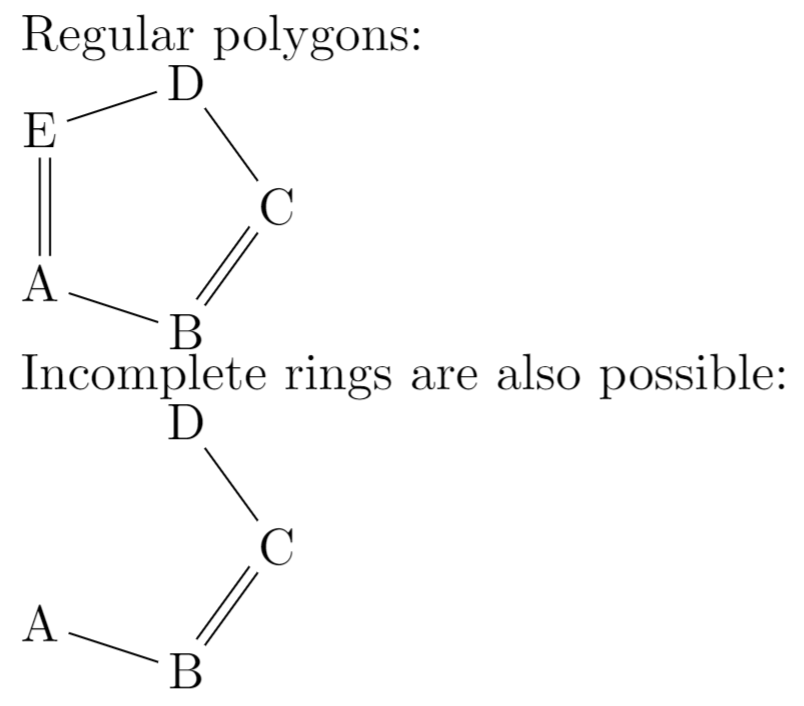The syntax of the command \chemfig{A*5(-B=C-D-E=)} is explained below: A: This is the first atom, the rest of the atoms will be drawn from here *5: Number of sides of the polygon (-B=C-D-E=): The rest of the atoms and bonds. If not enough are passed to complete the polygon, an incomplete ring will be drawn. There is an additional parameter that can be passed to this command: a double asterisk. For instance, if **5 is set instead of *5 a circle is drawn inside the polygon. Branches Chemical formulae are not always linear, branched formulae are actually the most common type. Below an example on how to create them. Branched molecule \vspace{.5cm} \chemfig{H-C(-H)(-H)-C(=O)-H}  Open this chemfig example in Overleaf The following image shows the output produced by the example above: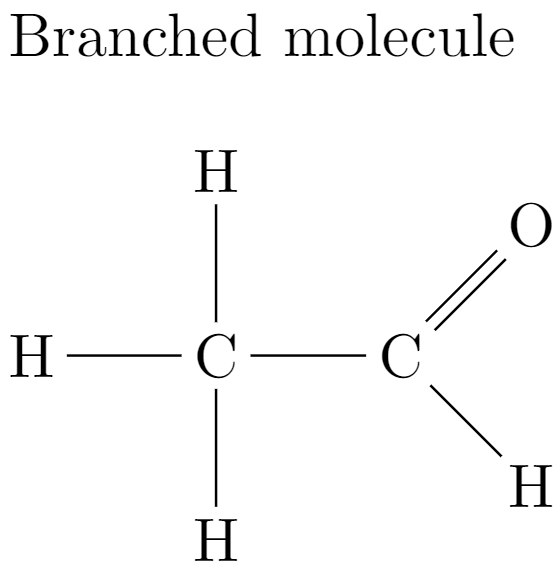Branches in each node are created by adding formulas inside parentheses. For instance, the code C(-H)(-H) creates two branches in "C", one with a 2 units angle (90°) and other with a 6 units angle (270°). Branches can also be added to rings: Branched ring \vspace{.5cm} \chemfig{A*6(-B=C(-CH_3)-D-E-F(=G)=)}  Open this chemfig example in Overleaf The following image shows the output produced by the example above: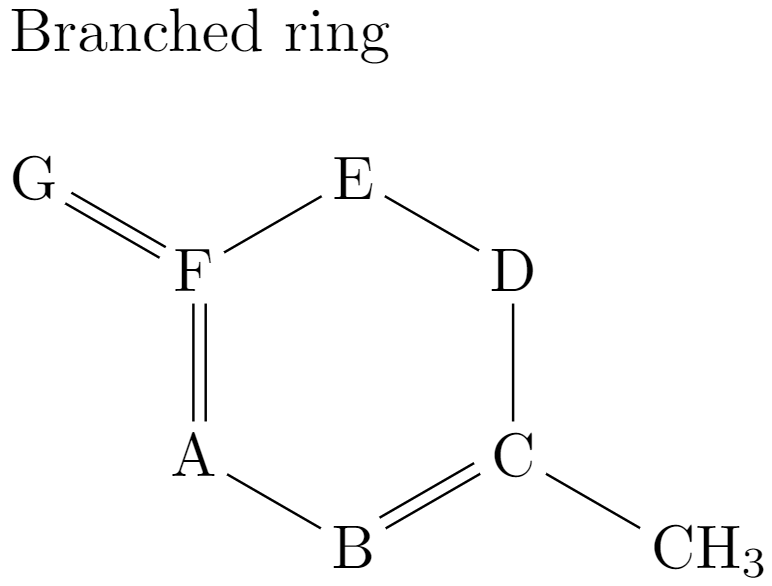The syntax is similar, using parentheses a branch can be attached to a node (atom). For instance F(=G) attaches a branch to the node "F". More complex examples can be created using nested branches and even attaching rings as branches. Customizing the formulae Several parameters such as colours and the node separation can be changed, also additional text to describe the formula can be added. {\huge \setchemfig{atom sep=2em,bond style={line width=1pt,red,dash pattern=on 2pt off 2pt}} \chemname {\chemfig{H-C(-H)(-H)-C(=O)-H}} {Ethanal} }  Open this chemfig example in Overleaf The following image shows the output produced by the example above: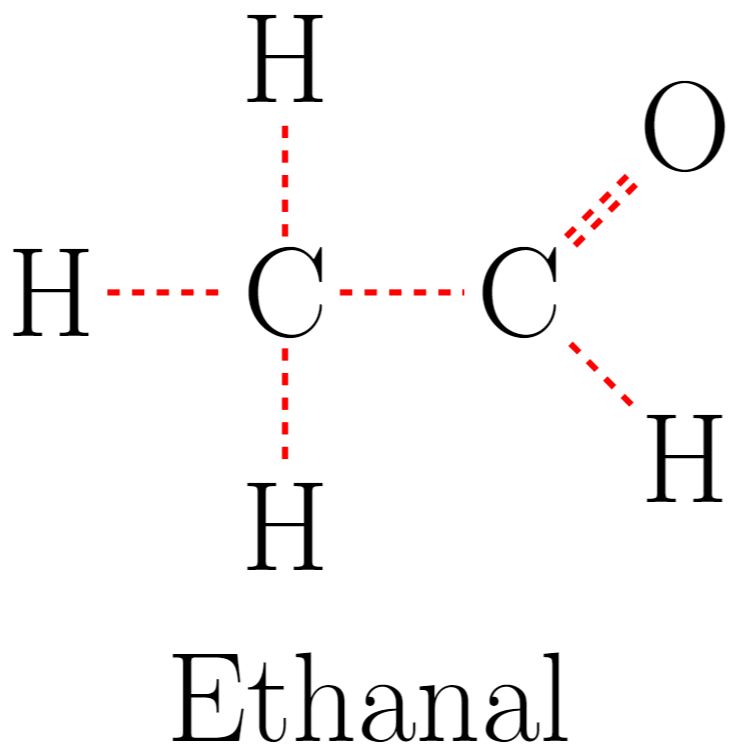There are three new command here: \setbondstyle{ }: Inside the braces several style-related commands can be passed using the H}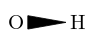plain left cram \chemfig{O < H}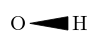dashed right cram \chemfig{O >: H}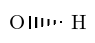dashed left cram \chemfig{O <: H}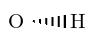hollow right cram \chemfig{O >| H}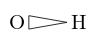hollow left cram \chemfig{O <| H}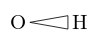Further reading For more information see Molecular orbital diagrams Feynman diagrams TikZ package Drawing Diagrams Directly in LaTeX Inserting Images List of Greek letters and math symbols The chemfig package documentation The tikz/pgf package documentation